# FAQs

#### UMS solves rational equations, which is a relatively simple task. Indeed, they can all be solved by essentially one method. What novelty does UMS bring to this task?

This task is not quite so simple. There are in fact many methods for solving rational equations, and about a hundred of these methods are used in our program. Try, for example to solve the following equations:
x4=(x+1)(5x2-6x-6)
(1+x+x2)4=(1+x2+x4)2
(x2+1)2(x+1)=0.8
UMS solves rational, irrational, logarithmic, exponential and trigonometric equations.

#### How can I enter the simultaneous equations?

To input the simultaneous equations, click the { button and enter each of the equations on a separate line. To add the next equation, press the Enter button.

#### I attempted to simplify the problem (a+b)2 by using the “Expressions simplification” section and got the same expression as the result. Why?

The UMS program considers this expression to already be in the simplest possible form. We recommend you select “Expand” in the “Mathematical Subject” menu.

#### I entered the problem fx = x2 – x3 and wanted to graph the function, but UMS thinks this is an equation and solves for the equation with parameters. Why?

The argument of the entered function must be enclosed in parentheses. For example f(x) = x2 – x3 or y(x) = x2 – x3, or the argument can be omitted, for example y = x2 – x3. In this case UMS would consider the expression as a function.

#### I selected the “Factoring” section and entered the problem x4-y4 and got (x2-y2)(x2+y2) as a result. But (x2-y2) can be decomposed into factors (x-y)(x+y). Why didn’t UMS finish the job?

We recommend you select “Complete factoring” in the “Mathematical Subject” menu.

#### I entered the problem 58972356356x3+23489755x7=357, and UMS did not solve it. Why?

This example does not allow for a precise solution. UMS is designed to find precise results (perhaps in radicals), but not approximate ones.

#### I want to use UMS to solve a problem such as: Find all values of parameter a so that both roots of the quadratic equation x2– 6ax + (2-2a+9a2)=0 are greater than 3.

You need to use the quadratic function y = x2 + px + q: The graph of this function is a parabola with branches pointing up.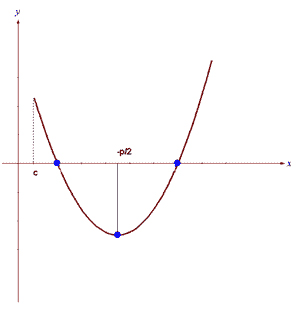The Vertex of the parabola has an x-axis value of x = – p/2. In your example p = – 6a, x= – p/2 = 3a. In order for both roots of the quadratic function to be greater than some number c, it is necessary and sufficient for the system of three inequalities to be true: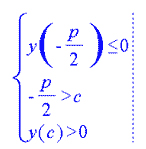In your example this system will look like this: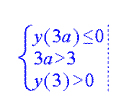Or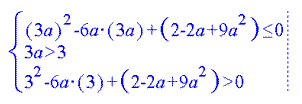This system of inequalities can be solved using UMS.

#### I entered the problem log(x) + log(x)2 + log(x)4 = 7, and UMS did not solve it. Why? This seems to be a simple equation.

The equation was not written correctly. In your equation log(x) is raised to the square and to the fourth degree. If we write this equation in the form: log(x) + log(x2) + log(x4) = 7, then argument x is raised to the square and to the fourth degree. Now UMS can solve this simple equation.

#### I want to use UMS to find P(x) = x2 – 60x +76 for x=45. I entered P(45) = x2 – 60x +76, but UMS did not solve it. Why didn’t UMS find P(45)?

In order to find the value F(c) of a function F=f(x) for the given value of variable x=c enter the following system and solve it with UMS.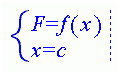In your example this system will look like this: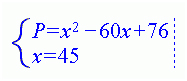UMS solves this system and receives: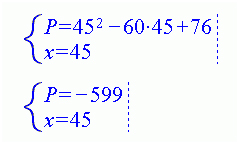that is P(45) = -599

#### I want to use UMS to find sin of 210 degrees. I entered sin(210), but UMS did not solve it. UMS Calculator gives the wrong answer! Why?

You forgot to enter the degree sign. You must enter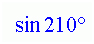Now UMS solves this problem and gives the right answer -1/2.

Free Algebra Equation Solver is our regular Universal Math Solver software with “Equations” section enabled for free. It does not require a license or any type of registration whatsoever in order to solve your equations and systems of equations. However, if you try to solve a user defined problem from other topics like for example Inequalities or Factoring it will ask for a license. You can still demo step by step solutions from other sections by selecting a problem from the main Collection. Please make sure you enter equations or systems of equations only if you want to use the free version.

#### I installed Universal Math Solver software but it does not start.

You may have to re-install the UMS software using Administrator account. Once it is installed, you can run it under a regular User account.

#### I found a crack for the full version of Universal Math Solver on internet.

We assure you that there are no valid cracks for the Universal Math Solver software. Best case scenario you will get a number that is actually a valid license. It may or may not work depending on that license expiration date and number of prior installations. Worst case scenario you will get a virus. Please download only from our site, CNET Download site or Software Informer site. Free Algebra Equation Solver is free as the name implies and does not require any license or code.

For Windows OS